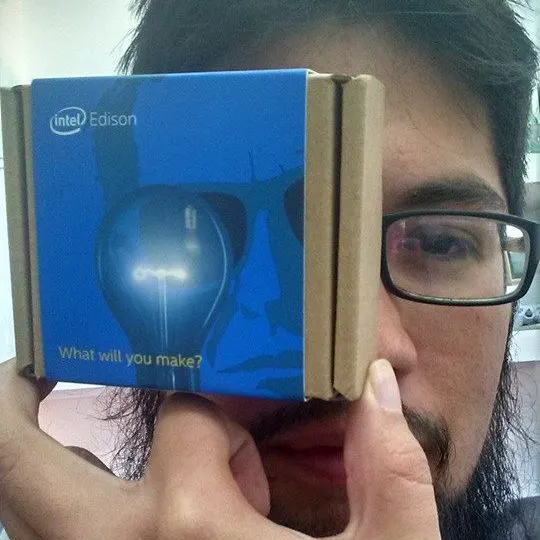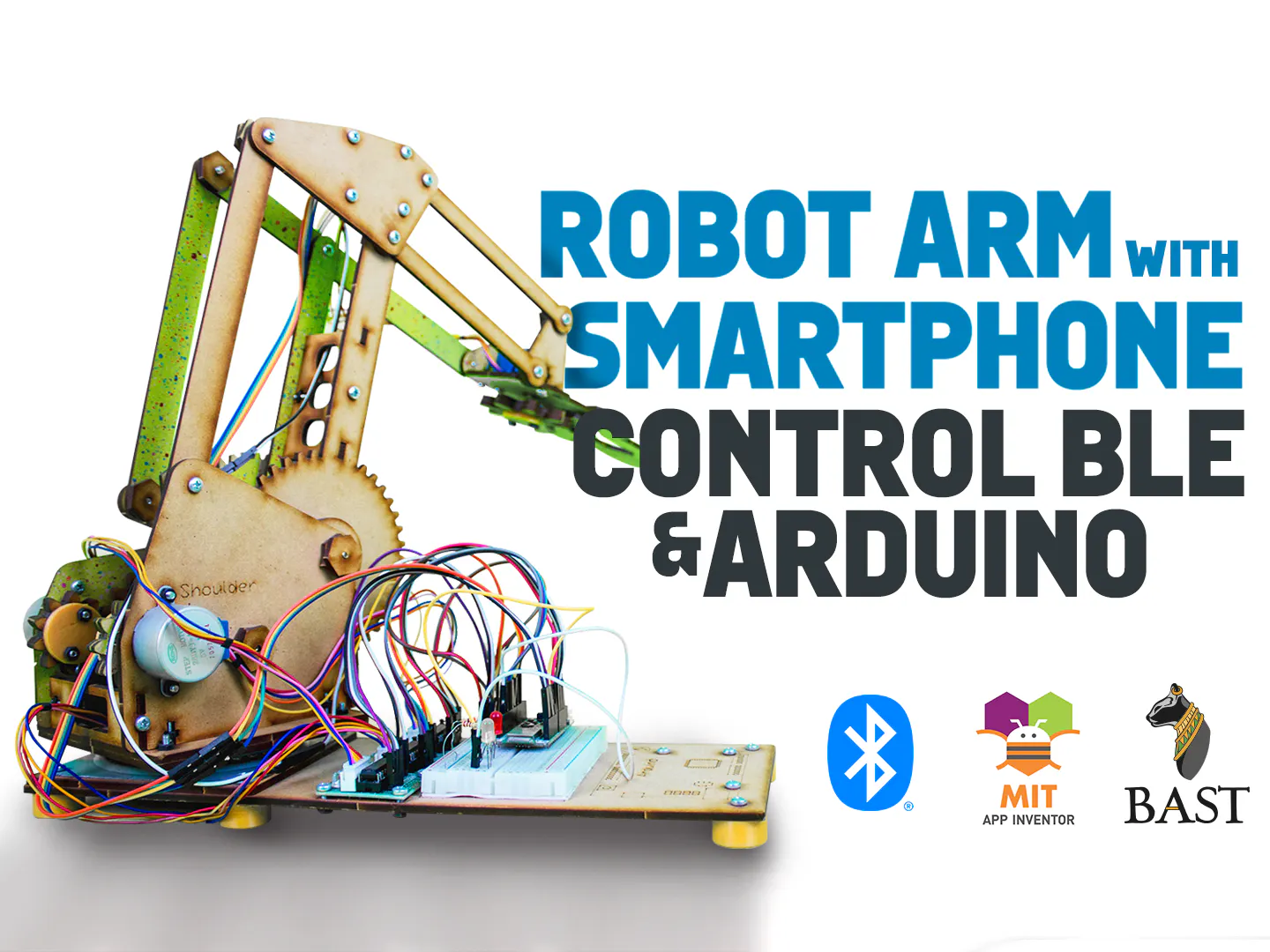# Robot Arm with Smartphone Control BLE and Arduino

Are you thinking about make an Android app for arm robot with BLE? With this project you can understand how to work BLE and control it.

IntermediateFull instructions provided5 hours499## Things used in this project

### Hardware components

 Bast BLE
×1SparkFun Pushbutton switch 12mm
×2LED (generic)
×3
 Robotic Arm
×1

### Software apps and online servicesMIT App Inventor 2Arduino IDE

## Code

### App Control Arm Robot BLE

Java
APK for Android System
`No preview (download only).`

### Firmware Control Robot BLE Arduino

Arduino
```int a;
int b;
int c;
#include <ArduinoBLE.h>
int x;
int y;
static const char* greeting = "Hello World!";
BLEService greetingService("180C");
int dato;
BLEService ledService("19B10000-E8F2-537E-4F6C-D104768A1214");

int paso  =
{
{1, 1, 0, 0},
{0, 1, 1, 0},
{0, 0, 1, 1},
{1, 0, 0, 1}
};

int paso2  =
{
{1, 1, 0, 0},
{0, 1, 1, 0},
{0, 0, 1, 1},
{1, 0, 0, 1}
};
int paso3  =
{
{1, 1, 0, 0},
{0, 1, 1, 0},
{0, 0, 1, 1},
{1, 0, 0, 1}
};
int paso4  =
{
{1, 1, 0, 0},
{0, 1, 1, 0},
{0, 0, 1, 1},
{1, 0, 0, 1}
};

void setup() {
while (!Serial);
Serial.begin(115200);
for (x = 2; x <= 13; x++) {
pinMode(x, OUTPUT);
}
for (x = 15; x <= 18; x++) {
pinMode(x, OUTPUT);
}
if (!BLE.begin()) {
Serial.println("starting BLE failed!");
while (1);
}
BLE.setLocalName("bast ble ec");
greetingCharacteristic.setValue(greeting);
Serial.print("Peripheral device MAC: ");
Serial.println("esperando coneccion...");

switchCharacteristic.writeValue(0);
}

void loop() {
BLEDevice central = BLE.central();
if (central) {
Serial.print("Connected to central MAC: ");
}
digitalWrite(8, HIGH);
while (central.connected()){
if (y == 0){
if (switchCharacteristic.written()) {
if (switchCharacteristic.value() == 'O' ) {   // any value other than 0
Serial.println("GRIPER OPEN");
for (x = 0; x < 15; x++) {
for (int i = 0; i < 4; i++)
{
digitalWrite(15, paso[i]);
digitalWrite(16, paso[i]);
digitalWrite(17, paso[i]);
digitalWrite(18, paso[i]);
delay(5);
}
}
} else if (switchCharacteristic.value() == 'C' ) {   // any value other than 0
Serial.println("GRIPER CLOCED");
for (x = 0; x < 15; x++) {
for (int i = 3; i >= 0; i--)
{
digitalWrite(15, paso[i]);
digitalWrite(16, paso[i]);
digitalWrite(17, paso[i]);
digitalWrite(18, paso[i]);
delay(5);
}
}
} else if (switchCharacteristic.value() == 'U' ) {                              // a 0 value
Serial.println("ARM UP");
for (x = 0; x < 15; x++) {
for (int i = 0; i < 4; i++)
{
digitalWrite(2, paso2[i]);
digitalWrite(3, paso2[i]);
digitalWrite(5, paso2[i]);
digitalWrite(6, paso2[i]);
delay(5);
}
}

}else if (switchCharacteristic.value() == 'D' ) {                              // a 0 value
Serial.println("ARM DOWN");
for (x = 0; x < 15; x++) {
for (int i = 3; i >= 0; i--)
{
digitalWrite(2, paso2[i]);
digitalWrite(3, paso2[i]);
digitalWrite(5, paso2[i]);
digitalWrite(6, paso2[i]);
delay(5);
}
}
}else if (switchCharacteristic.value() == 'A' ) {                              // a 0 value
Serial.println("BOW UP");
for (x = 0; x < 15; x++) {
for (int i = 0; i < 4; i++)
{
digitalWrite(10, paso3[i]);
digitalWrite(11, paso3[i]);
digitalWrite(12, paso3[i]);
digitalWrite(13, paso3[i]);
delay(5);
}
}
}else if (switchCharacteristic.value() == 'B' ) {                              // a 0 value
Serial.println("BOW DOWN");
for (x = 0; x < 15; x++) {
for (int i = 3; i >= 0; i--)
{
digitalWrite(10, paso3[i]);
digitalWrite(11, paso3[i]);
digitalWrite(12, paso3[i]);
digitalWrite(13, paso3[i]);
delay(5);
}
}
}else if (switchCharacteristic.value() == 'L' ) {                              // a 0 value
Serial.println("BASE LEFT");
for (x = 0; x < 15; x++) {
for (int i = 0; i < 4; i++)
{
digitalWrite(19, paso4[i]);
digitalWrite(20, paso4[i]);
digitalWrite(4, paso4[i]);
digitalWrite(7, paso4[i]);
delay(5);
}
}
}else if (switchCharacteristic.value() == 'R' ) {                              // a 0 value
Serial.println("BASE RIGTH");
for (x = 0; x < 15; x++) {
for (int i = 3; i >= 0; i--)
{
digitalWrite(19, paso4[i]);
digitalWrite(20, paso4[i]);
digitalWrite(4, paso4[i]);
digitalWrite(7, paso4[i]);
delay(5);
}
}
}else if (switchCharacteristic.value() == 'G' ) {
// a 0 value
y = 1;
b = 0;
Serial.println(F("RECORDING MOVIMENTS"));
}else if (switchCharacteristic.value() == 'P' ) {
// a 0 value
y = 2;
b = 0;
Serial.println("RECORDING MOVIMENTS");
}
}
}
if (y == 1) {
if (switchCharacteristic.written()) {
if (switchCharacteristic.value() == 'O' ) {   // any value other than 0
Serial.println("GRIPER OPEN");
a[b] = 'O';
b++;
for (x = 0; x < 15; x++) {
for (int i = 0; i < 4; i++)
{
digitalWrite(15, paso[i]);
digitalWrite(16, paso[i]);
digitalWrite(17, paso[i]);
digitalWrite(18, paso[i]);
delay(5);
}
}
} else if (switchCharacteristic.value() == 'C' ) {   // any value other than 0
Serial.println("GRIPER CLOCE");
a[b] = 'C';
b++;
for (x = 0; x < 15; x++) {
for (int i = 3; i >= 0; i--)
{
digitalWrite(15, paso[i]);
digitalWrite(16, paso[i]);
digitalWrite(17, paso[i]);
digitalWrite(18, paso[i]);
delay(5);
}
}
} else if (switchCharacteristic.value() == 'U' ) {                              // a 0 value
Serial.println("ARM UP");
a[b] = 'U';
b++;
for (x = 0; x < 15; x++) {
for (int i = 0; i < 4; i++)
{
digitalWrite(2, paso2[i]);
digitalWrite(3, paso2[i]);
digitalWrite(5, paso2[i]);
digitalWrite(6, paso2[i]);
delay(5);
}
}

}else if (switchCharacteristic.value() == 'D' ) {                              // a 0 value
Serial.println("ARM DOWN");
a[b] = 'D';
b++;
for (x = 0; x < 15; x++) {
for (int i = 3; i >= 0; i--)
{
digitalWrite(2, paso2[i]);
digitalWrite(3, paso2[i]);
digitalWrite(5, paso2[i]);
digitalWrite(6, paso2[i]);
delay(5);
}
}
}else if (switchCharacteristic.value() == 'A' ) {                              // a 0 value
Serial.println("BOW UP");
a[b] = 'A';
b++;
for (x = 0; x < 15; x++) {
for (int i = 0; i < 4; i++)
{
digitalWrite(10, paso3[i]);
digitalWrite(11, paso3[i]);
digitalWrite(12, paso3[i]);
digitalWrite(13, paso3[i]);
delay(5);
}
}
}else if (switchCharacteristic.value() == 'B' ) {                              // a 0 value
Serial.println("BOW DOWN");
a[b] = 'B';
b++;
for (x = 0; x < 15; x++) {
for (int i = 3; i >= 0; i--)
{
digitalWrite(10, paso3[i]);
digitalWrite(11, paso3[i]);
digitalWrite(12, paso3[i]);
digitalWrite(13, paso3[i]);
delay(5);
}
}
}else if (switchCharacteristic.value() == 'L' ) {                              // a 0 value
Serial.println("BASE LEFT");
a[b] = 'L';
b++;
for (x = 0; x < 15; x++) {
for (int i = 0; i < 4; i++)
{
digitalWrite(19, paso4[i]);
digitalWrite(20, paso4[i]);
digitalWrite(4, paso4[i]);
digitalWrite(7, paso4[i]);
delay(5);
}
}
}else if (switchCharacteristic.value() == 'R' ) {                              // a 0 value
Serial.println("BASE RIGTH");
a[b] = 'R';
b++;
for (x = 0; x < 15; x++) {
for (int i = 3; i >= 0; i--)
{
digitalWrite(19, paso4[i]);
digitalWrite(20, paso4[i]);
digitalWrite(4, paso4[i]);
digitalWrite(7, paso4[i]);
delay(5);
}
}
}else if (switchCharacteristic.value() == 'S' ) {
// a 0 value
c = b-1;
y = 0;
Serial.println(F("RECORDING MOVIMENTS"));
}
}
}
if (y ==2 ){
for (b=0; b<=c;b++)
{
a[b];
if (a[b]== 'O' ){
for (x = 0; x < 15; x++) {
for (int i = 0; i < 4; i++)
{
digitalWrite(15, paso[i]);
digitalWrite(16, paso[i]);
digitalWrite(17, paso[i]);
digitalWrite(18, paso[i]);
delay(5);
}
}
}
if (a[b]== 'C' ){
for (x = 0; x < 15; x++) {
for (int i = 3; i >= 0; i--)
{
digitalWrite(15, paso[i]);
digitalWrite(16, paso[i]);
digitalWrite(17, paso[i]);
digitalWrite(18, paso[i]);
delay(5);
}
}
}
if (a[b]== 'U' ){
for (x = 0; x < 15; x++) {
for (int i = 0; i < 4; i++)
{
digitalWrite(2, paso2[i]);
digitalWrite(3, paso2[i]);
digitalWrite(5, paso2[i]);
digitalWrite(6, paso2[i]);
delay(5);
}
}
}
if (a[b]== 'D' ){
for (x = 0; x < 15; x++) {
for (int i = 3; i >= 0; i--)
{
digitalWrite(2, paso2[i]);
digitalWrite(3, paso2[i]);
digitalWrite(5, paso2[i]);
digitalWrite(6, paso2[i]);
delay(5);
}
}
}
if (a[b]== 'A' ){
for (x = 0; x < 15; x++) {
for (int i = 0; i < 4; i++)
{
digitalWrite(10, paso3[i]);
digitalWrite(11, paso3[i]);
digitalWrite(12, paso3[i]);
digitalWrite(13, paso3[i]);
delay(5);
}
}
}
if (a[b]== 'B' ){
for (x = 0; x < 15; x++) {
for (int i = 3; i >= 0; i--)
{
digitalWrite(10, paso3[i]);
digitalWrite(11, paso3[i]);
digitalWrite(12, paso3[i]);
digitalWrite(13, paso3[i]);
delay(5);
}
}
}
if (a[b]== 'L' ){
for (x = 0; x < 15; x++) {
for (int i = 0; i < 4; i++)
{
digitalWrite(19, paso4[i]);
digitalWrite(20, paso4[i]);
digitalWrite(4, paso4[i]);
digitalWrite(7, paso4[i]);
delay(5);
}
}
}
if (a[b]== 'R' ){
for (x = 0; x < 15; x++) {
for (int i = 3; i >= 0; i--)
{
digitalWrite(19, paso4[i]);
digitalWrite(20, paso4[i]);
digitalWrite(4, paso4[i]);
digitalWrite(7, paso4[i]);
delay(5);
}
}
}
}
for (b=c; b>=0;b--)
{
a[b];
if (a[b]== 'O' ){
for (x = 0; x < 15; x++) {
for (int i = 3; i >= 0; i--)
{
digitalWrite(15, paso[i]);
digitalWrite(16, paso[i]);
digitalWrite(17, paso[i]);
digitalWrite(18, paso[i]);
delay(5);
}
}
}
if (a[b]== 'C' ){
for (x = 0; x < 15; x++) {
for (int i = 0; i < 4; i++)
{
digitalWrite(15, paso[i]);
digitalWrite(16, paso[i]);
digitalWrite(17, paso[i]);
digitalWrite(18, paso[i]);
delay(5);
}
}
}
if (a[b]== 'U' ){
for (x = 0; x < 15; x++) {
for (int i = 3; i >= 0; i--)
{
digitalWrite(2, paso2[i]);
digitalWrite(3, paso2[i]);
digitalWrite(5, paso2[i]);
digitalWrite(6, paso2[i]);
delay(5);
}
}
}
if (a[b]== 'D' ){
for (x = 0; x < 15; x++) {
for (int i = 0; i < 4; i++)
{
digitalWrite(2, paso2[i]);
digitalWrite(3, paso2[i]);
digitalWrite(5, paso2[i]);
digitalWrite(6, paso2[i]);
delay(5);
}
}
}
if (a[b]== 'A' ){
for (x = 0; x < 15; x++) {
for (int i = 3; i >= 0; i--)
{
digitalWrite(10, paso3[i]);
digitalWrite(11, paso3[i]);
digitalWrite(12, paso3[i]);
digitalWrite(13, paso3[i]);
delay(5);
}
}
}
if (a[b]== 'B' ){
for (x = 0; x < 15; x++) {
for (int i = 0; i < 4; i++)
{
digitalWrite(10, paso3[i]);
digitalWrite(11, paso3[i]);
digitalWrite(12, paso3[i]);
digitalWrite(13, paso3[i]);
delay(5);
}
}
}
if (a[b]== 'L' ){
for (x = 0; x < 15; x++) {
for (int i = 3; i >= 0; i--)
{
digitalWrite(19, paso4[i]);
digitalWrite(20, paso4[i]);
digitalWrite(4, paso4[i]);
digitalWrite(7, paso4[i]);
delay(5);
}
}
}
if (a[b]== 'R' ){
for (x = 0; x < 15; x++) {
for (int i = 0; i < 4; i++)
{
digitalWrite(19, paso4[i]);
digitalWrite(20, paso4[i]);
digitalWrite(4, paso4[i]);
digitalWrite(7, paso4[i]);
delay(5);
}
}

if (switchCharacteristic.written()) {
if (switchCharacteristic.value() == 'S' ) {
y=0;
b=0;
}
}

}
}
}
}
digitalWrite(8, LOW);
Serial.print("Disconnected from central MAC: ");